## Example Questions

### Example Question #1 : How To Find The Area Of A Pentagon

The perimeter of a regular pentagon is 40 units. What is the area of the pentagon?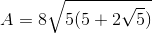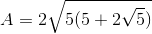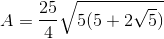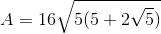Explanation:

The formula for the area of a regular pentagon is given by the equation: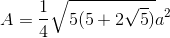where a is represented by the length of one side. Let's begin by finding the side length of the regular pentagon. If the perimeter is 40, then we can divide by 5 (the number of sides) to find a side length of 8.

If we plug in 8 into the equation: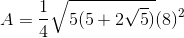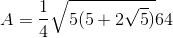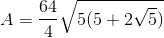Tired of practice problems?

Try live online GRE Math prep today.